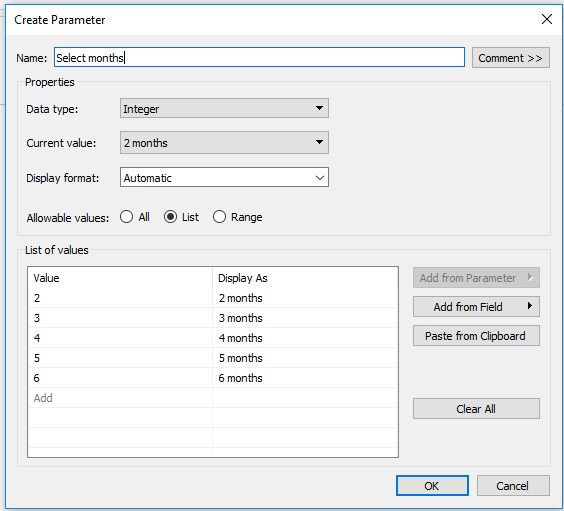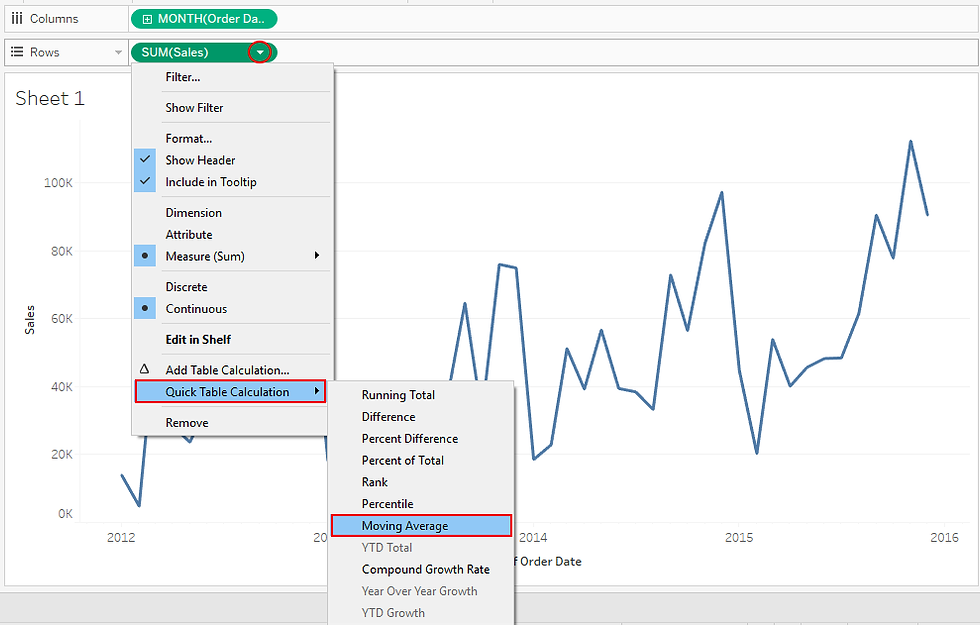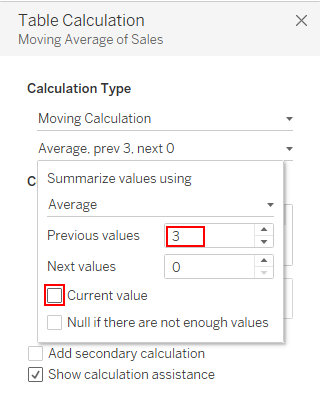top of page
Search

# Tableau Tips: Parameters - Table Calculations

Updated: Aug 18, 2021In the previous articles on application of parameters, we explored how we can apply parameters with sets, calculated fields, bins and reference line. In this article, we would like to explore the fifth use case of parameters with table calculations.

I know, somebody might be thinking, how is this possible? Hold on, because there is a catch somewhere. But, be sure by the end of this article you’ll learn the catch to using parameters with table calculations.

### Our problem

In this hypothetical case, we assume the position of data analysts at Superstores chain, where our manager has tasked us to compute the moving average for the Sales in 3 months prior to a certain month, let’s say June 2015.

### Our Solution

Experience tells us that the manager Superstore is unpredictable, therefore his demands can change any time, by the time the report is ready, maybe his interest will be moving average in 2, 4 or 6 months prior to June 2015 and not the three months requested during the project’s inception.

Therefore, for us to be prepared for any unexpected changes, we can use parameter with table calculations to build an interface which enables the manager to glean insights in case his question changes.

Using Superstores data sets pre-packaged with Tableau app.

### Create parameterLet’s build our view;

• Drag dimension field ‘Order Date’ to the columns shelf, adjust the level of detail to month/year - continuous fields.

• Drag measure field ‘Sales’ to the rows shelf.

• Add a Quick Table Calculation > Moving Average.Edit table calculation;Use the three prior values, not including the current value. (This way the June 2015 value will include average of the three prior months. (March, April, May).Mouse-over June 2015 we’ve.This gives the solutions we’ve been tasked, but it’s rigid and doesn’t give the manager flexibility to test other hypothesis. e.g. moving average in 2, 4, 5, or 6 months prior to June 2015.

### Apply parameter with the table calculation

To effect this;

Hold down control key and drag table calculation SUM(Sales) from the rows shelf to the data pane window.

Note: This creates a copy of the table calculation inform of a calculated field named ‘Calculation1Rename it ‘Moving Average

Edit the field ‘Moving Average’.On the dialog box, edit the calculation by replacing 3 with our parameter ‘Select months’.Replace the table calculation on the rows shelf SUM(Sales) with our field ‘Moving Average’.

Show the Parameter control.With this view, the manager of Superstore can easily choose the level of computation in the view.

### What was the catch to this technique?

To use parameters with table calculations, you must convert the table calculation to a basic calculation, or simply use the calculated field editor to write your table calculation, this gives more control on which fields (dimension, measure or parameter) to use in your table calculation.

### What next?

In the next and last article on parameters, we’ll explore on how parameters can be used to filter across different data sources.

Note: You can revisit the previous use cases of parameters with, bins, calculated field, reference lines and sets to learn more.

To receive more of the Tableau tips and tricks, kindly join our mailing list by subscribing below.